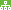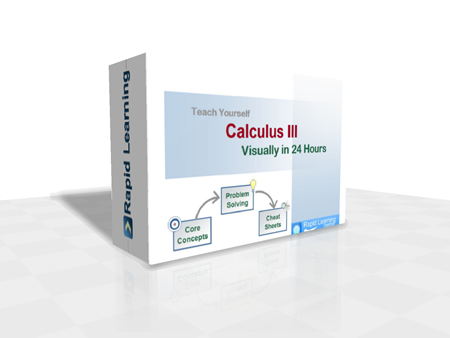How to Learn in 24 Hours?The Rapid Learning Movie

 Need Help? M-F: 9am-5pm(PST): Toll-Free: (877) RAPID-10 US Direct: (714) 692-2900 Int'l: 001-714-692-2900 24/7 Online Technical Support: The Rapid Support Center Secure Online Order:Need Proof? Testimonials by Our Users

 Rapid Learning Courses: Chemistry in 24 Hours Biology in 24 Hours Physics in 24 Hours Mathematics in 24 Hours Psychology in 24 Hours SAT in 24 Hours ACT in 24 Hours AP in 24 Hours CLEP in 24 Hours MCAT in 24 Hours (Medical) USMLE in 24 Hours (Boards) DAT in 24 Hours (Dental) OAT in 24 Hours (Optometry) PCAT in 24 Hours (Pharmacy) Nursing Entrance Exams Certification in 24 Hours eBook - Survival Kits Audiobooks (MP3)

 Tell-A-Friend: Have friends taking science and math courses too? Tell them about our rapid learning system.Home »  Mathematics » Calculus IIICourse Preview

Calculus III Rapid Learning Series is the 3rd of the three-course calculus sequence (I, II & III), designed typically for math, science and engineering majors or anyone who is required to take three semesters of calculus courses in college. This sequence of three courses cover the single variable and multivariable calculus. If you are required to take only one semester of calculus course, go for our College Calculus - RL411. If you are required to take three semesters of calculus courses, you should take our Calculus I, Calculus II and Calculus III, each represents one-semester of contents sequentially.

Calculus is the study of how things change. In a first Calculus course, you learn topics such as Limits, Differentiation, and Integration. In Calculus II, you extend your knowledge of Calculus to other types of functions and will learn additional integration and differentiation techniques. In this Calculus III, you will generalize previous techniques to vectors and functions with multiple variables. This means that you are often dealing with higher dimensional objects than before.

1. 24 Core Concept Tutorials (Flash Movies)
24x visual tutorials for 24 chapters, with concept map, concept illustration, step-wise problem solving and topical summary, narrated by college calculus professors.
2. 24 Problem-Solving Drills (Flash Games)
24x feedback based Quizzes, concept-based problems, summary reviews on all problems and scoring system to track performance.
3. 24 Super Review Cheat Sheets (PDF Printables)
One cheat sheet per topic, key concepts in a single-sheet, at-a-glance review of each topic and both printable and laminatible Ideal for exam prep quick review.
Pre-requisites for Calculus: (1) Elementary Algebra - RL409 (2) Intermediate Algebra - RL407 (3) Pre-Calculus - RL403.

Calculus III Rapid Learning Series

This 24-chapter series provides comprehensive coverage of traditional college calculus topics for any students who are taking multiple semester calculus courses.

Core Unit #1 – The Foundation

• Tutorial 01: Introduction to Calculus III
• Tutorial 02: Precalculus Review
• Tutorial 03: Review of Calculus I
• Tutorial 04: Review of Calculus II

Core Unit #2 – Vectors, Curves and Surfaces in Space

• Tutorial 05: Space Coordinates and Vectors in the Plane and Space
• Tutorial 06: The Dot Product and the Cross Product
• Tutorial 07: Lines and Planes in Space
• Tutorial 08: Cylindrical and Quadric Surfaces

Core Unit #3 – Vector-Valued Functions

• Tutorial 09: Vector Functions, Space Curves, Calculus of Vector Functions, Velocity and Acceleration
• Tutorial 10: Arc Length, Unit Tangent Vector, Curvature and Unit Normal Vector

Core Unit #4 – Partial Derivatives

• Tutorial 11: Limits and Continuity of Functions of Several Variables
• Tutorial 12: Partial Derivatives
• Tutorial 13: The Chain Rule
• Tutorial 14: Tangent Planes and Linear Approximation
• Tutorial 15: Directional Derivatives and the Gradient Vector
• Tutorial 16: Extrema of Two Variable Functions and Lagrange Multipliers

Core Unit #5 – Multiple Integrals

• Tutorial 17: Iterated and Double Integrals
• Tutorial 18: Double Integrals in Rectangular and Polar Coordinates, and Applications
• Tutorial 19: Triple Integrals and Applications
• Tutorial 20: Triple Integrals in Cylindrical and Spherical Coordinates

Core Unit #6 – Vector Calculus

• Tutorial 21: Vector Fields, Line Integrals and Independence of Path
• Tutorial 22: Green's Theorem
• Tutorial 23: Surface Areas and Surface Integrals
• Tutorial 24: Stokes' Theorem and Divergence Theorem

Each chapter comes with five learning components:
(1) Rich-Media Tutorials (Standard, Premium and Platinum Edition)
(2) Problem-Solving Drills (Standard, Premium and Platinum Edition)
(3) Super-Review Cheatsheets (Standard, Premium and Platinum Edition)
(4) Printable Tutorials (Premium and Platinum Edition)
(5) MP3 Audio Lectures (Platinum Edition Only)

Master Calculus the rich-media way, get started now:

Course Preview